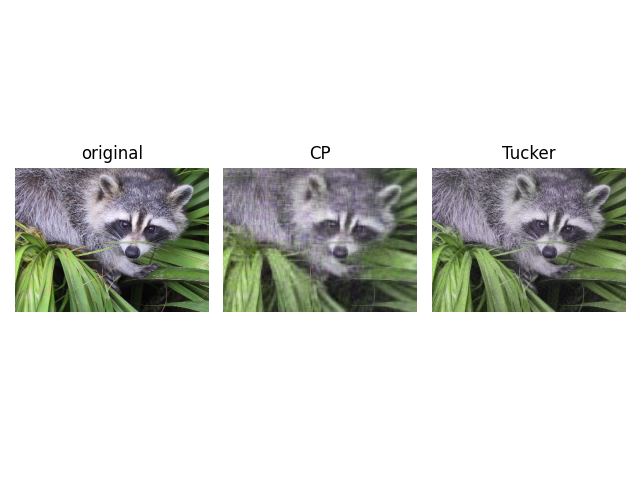# Image compression via tensor decomposition

Example on how to use `tensorly.decomposition.parafac` and `tensorly.decomposition.tucker` on images.```/home/runner/work/tensorly/tensorly/examples/applications/plot_image_compression.py:21: DeprecationWarning: scipy.misc.face has been deprecated in SciPy v1.10.0; and will be completely removed in SciPy v1.12.0. Dataset methods have moved into the scipy.datasets module. Use scipy.datasets.face instead.
image = face()
/home/runner/work/tensorly/tensorly/examples/applications/plot_image_compression.py:22: DeprecationWarning: scipy.misc.face has been deprecated in SciPy v1.10.0; and will be completely removed in SciPy v1.12.0. Dataset methods have moved into the scipy.datasets module. Use scipy.datasets.face instead.
image = tl.tensor(zoom(face(), (0.3, 0.3, 1)), dtype='float64')
```

```import matplotlib.pyplot as plt
import tensorly as tl
import numpy as np
from scipy.misc import face
from scipy.ndimage import zoom
from tensorly.decomposition import parafac
from tensorly.decomposition import tucker
from math import ceil

random_state = 12345

image = face()
image = tl.tensor(zoom(face(), (0.3, 0.3, 1)), dtype='float64')

def to_image(tensor):
"""A convenience function to convert from a float dtype back to uint8"""
im = tl.to_numpy(tensor)
im -= im.min()
im /= im.max()
im *= 255
return im.astype(np.uint8)

# Rank of the CP decomposition
cp_rank = 25
# Rank of the Tucker decomposition
tucker_rank = [100, 100, 2]

# Perform the CP decomposition
weights, factors = parafac(image, rank=cp_rank, init='random', tol=10e-6)
# Reconstruct the image from the factors
cp_reconstruction = tl.cp_to_tensor((weights, factors))

# Tucker decomposition
core, tucker_factors = tucker(image, rank=tucker_rank, init='random', tol=10e-5, random_state=random_state)
tucker_reconstruction = tl.tucker_to_tensor((core, tucker_factors))

# Plotting the original and reconstruction from the decompositions
fig = plt.figure()
ax.set_axis_off()
ax.imshow(to_image(image))
ax.set_title('original')

ax.set_axis_off()
ax.imshow(to_image(cp_reconstruction))
ax.set_title('CP')